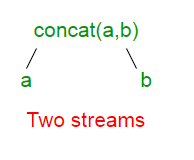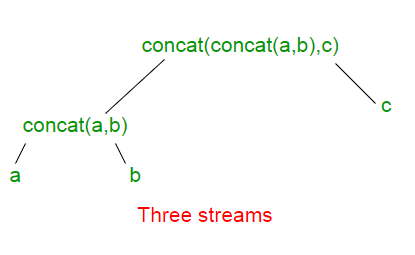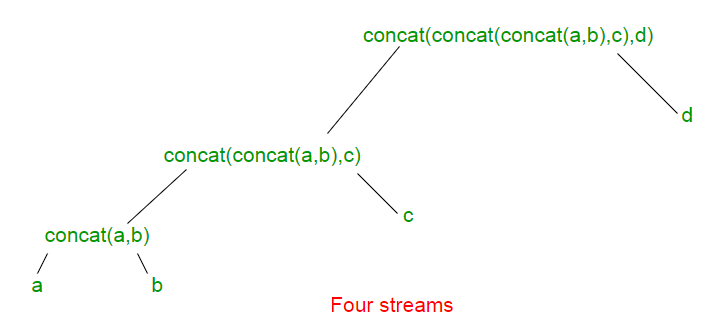# Stream.concat() in Java

Stream.concat() method creates a concatenated stream in which the elements are all the elements of the first stream followed by all the elements of the second stream. The resulting stream is ordered if both of the input streams are ordered, and parallel if either of the input streams is parallel.

Syntax :

```static <T> Stream<T> concat(Stream<? extends T> stream1,
Stream<? extends T> stream2)

Where, T is the type of stream elements,
stream1 represents the first stream,
stream2 represents the second stream and
the function returns the concatenation of
the two input streams
```

The calls to Stream.concat(stream1, stream2) can be think of as forming a binary tree. The concatenation of all the input streams is at the root. The individual input streams are at the leaves. Below given are some examples of trees upto four input streams a, b, c and d.

For two streams a and b, the tree looks like :For three streams a, b and c, the tree looks like :For four streams a, b, c and d, the tree looks like :Each additional input stream adds one layer of depth to the tree and one layer of indirection to reach all the other streams.

Note : The elements returned by Stream.concat() method is ordered. For example, the following two lines returns the same result:

```Stream.concat(Stream.concat(stream1, stream2), stream3);
Stream.concat(stream1, Stream.concat(stream2, stream3));
```

But the result for the following two are different.

```Stream.concat(Stream.concat(stream1, stream2), stream3);
Stream.concat(Stream.concat(stream2, stream1), stream3);
```

Below are some examples to understand the implementation of the function in a better way.
Example 1 :

 `// Implementation of Stream.concat() ` `// method in Java 8 with 2 Streams ` `import` `java.util.*; ` `import` `java.util.stream.IntStream; ` `import` `java.util.stream.Stream; ` ` `  `class` `GFG { ` ` `  `    ``// Driver code ` `    ``public` `static` `void` `main(String[] args) ` `    ``{ ` `        ``// Creating two Streams ` `        ``Stream stream1 = Stream.of(``"Geeks"``, ``"for"``); ` `        ``Stream stream2 = Stream.of(``"GeeksQuiz"``, ``"GeeksforGeeks"``); ` ` `  `        ``// concatenating both the Streams ` `        ``// with Stream.concat() function ` `        ``// and displaying the result ` `        ``Stream.concat(stream1, stream2) ` `            ``.forEach(element -> System.out.println(element)); ` `    ``} ` `} `

Output:

```Geeks
for
GeeksQuiz
GeeksforGeeks
```

Example 2 :

 `// Implementation of Stream.concat() ` `// method in Java 8 with more than ` `// two Streams ` `import` `java.util.*; ` `import` `java.util.stream.IntStream; ` `import` `java.util.stream.Stream; ` ` `  `class` `GFG { ` ` `  `    ``// Driver code ` `    ``public` `static` `void` `main(String[] args) ` `    ``{ ` ` `  `        ``// Creating more than two Streams ` `        ``Stream stream1 = Stream.of(``"Geeks"``); ` `        ``Stream stream2 = Stream.of(``"GeeksQuiz"``); ` `        ``Stream stream3 = Stream.of(``"GeeksforGeeks"``); ` `        ``Stream stream4 = Stream.of(``"GFG"``); ` ` `  `        ``// concatenating all the Streams ` `        ``// with Stream.concat() function ` `        ``// and displaying the result ` `        ``Stream.concat(Stream.concat(Stream.concat(stream1, ` `                             ``stream2), stream3), stream4) ` `            ``.forEach(element -> System.out.println(element)); ` `    ``} ` `} `

Output:

```Geeks
GeeksQuiz
GeeksforGeeks
GFG
```

Example 3 :

 `// Implementation of Stream.concat() ` `// method in Java 8 with DoubleStream ` `import` `java.util.*; ` `import` `java.util.stream.Stream; ` `import` `java.util.stream.DoubleStream; ` ` `  `class` `GFG { ` ` `  `    ``// Driver code ` `    ``public` `static` `void` `main(String[] args) ` `    ``{ ` ` `  `        ``// Creating two Streams ` `        ``DoubleStream Stream1 = DoubleStream.of(``1520``, ``1620``); ` `        ``DoubleStream Stream2 = DoubleStream.of(``1720``, ``1820``); ` ` `  `        ``// concatenating both the Streams and ` `        ``// displaying the result ` `        ``DoubleStream.concat(Stream1, Stream2) ` `            ``.forEach(element -> System.out.println(element)); ` `    ``} ` `} `

Output:

```1520.0
1620.0
1720.0
1820.0
```

Example 4 :

 `// Implementation of Stream.concat() ` `// method in Java 8 and removing ` `// the duplicates ` `import` `java.util.*; ` `import` `java.util.stream.IntStream; ` `import` `java.util.stream.Stream; ` ` `  `class` `GFG { ` ` `  `    ``// Driver code ` `    ``public` `static` `void` `main(String[] args) ` `    ``{ ` ` `  `        ``// Creating two Streams ` `        ``Stream stream1 = Stream.of(``"Geeks"``, ``"for"``, ``"GeeksforGeeks"``); ` `        ``Stream stream2 = Stream.of(``"GeeksQuiz"``, ``"GeeksforGeeks"``, ``"for"``); ` ` `  `        ``// concatenating both the Streams ` `        ``// with Stream.concat() function ` `        ``// and displaying the result after ` `        ``// removing the duplicates ` `        ``Stream.concat(stream1, stream2).distinct().forEach(element -> System.out.println(element)); ` `    ``} ` `} `

Output:

```Geeks
for
GeeksforGeeks
GeeksQuiz
```

Example 5 :

 `// Implementation of Stream.concat() ` `// method in Java 8 with LongStream ` `import` `java.util.*; ` `import` `java.util.stream.Stream; ` `import` `java.util.stream.LongStream; ` ` `  `class` `GFG { ` ` `  `    ``// Driver code ` `    ``public` `static` `void` `main(String[] args) ` `    ``{ ` ` `  `        ``// Creating two Streams ` `        ``LongStream Stream1 = LongStream.of(``1520``, ``1620``); ` `        ``LongStream Stream2 = LongStream.of(``1720``, ``1820``); ` ` `  `        ``// concatenating both the Streams and ` `        ``// displaying the result ` `        ``LongStream.concat(Stream1, Stream2) ` `            ``.forEach(element -> System.out.println(element)); ` `    ``} ` `} `

Output:

```1520
1620
1720
1820
```

My Personal Notes arrow_drop_upIn love with a semicolon because sometimes i miss it so badly)

If you like GeeksforGeeks and would like to contribute, you can also write an article using contribute.geeksforgeeks.org or mail your article to contribute@geeksforgeeks.org. See your article appearing on the GeeksforGeeks main page and help other Geeks.

Please Improve this article if you find anything incorrect by clicking on the "Improve Article" button below.

Article Tags :
Practice Tags :

Be the First to upvote.

Please write to us at contribute@geeksforgeeks.org to report any issue with the above content.